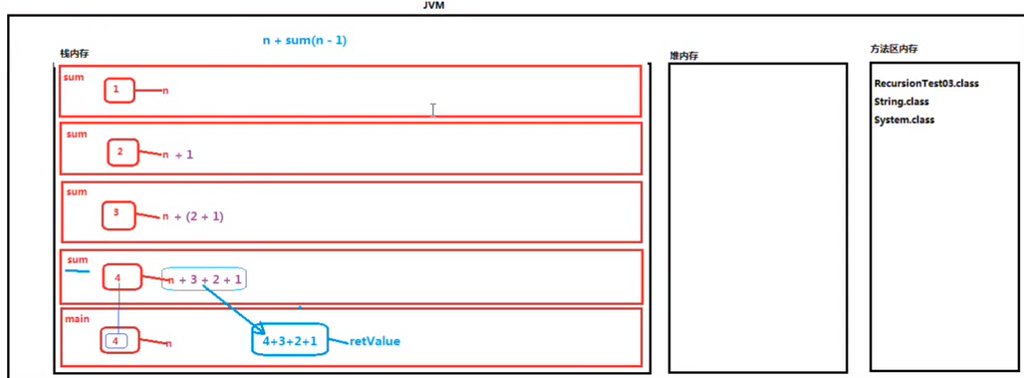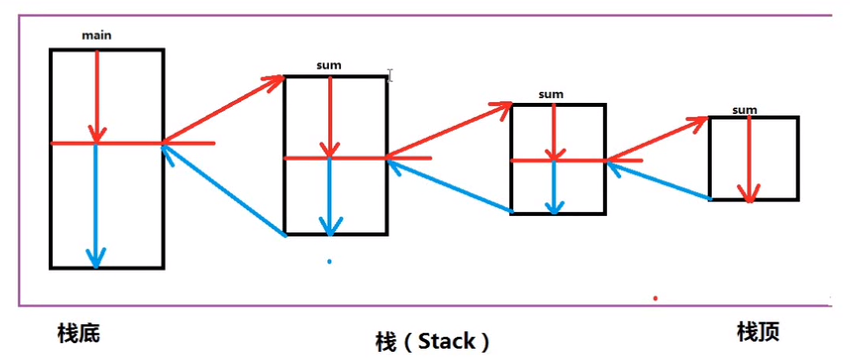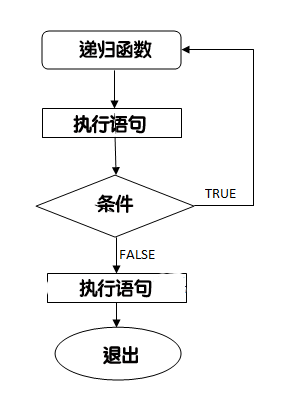• 下列属于递归调用的是
2020-11-24 09:14:07

递归一个通俗的解释就是，在函数中调用函数本身；伪代码如下：

In : def fun():

....: fun()

# 这个递归没有任何作用，只是为了说明什么是递归

递归（Recursion），在数学与计算机科学中，是指在函数的定义中使用函数自身的方法。

在使用递归时，需要注意以下几点：

递归就是在过程或函数里调用自身

必须有一个明确的递归结束条件，称为递归出口。

注意： 切勿忘记递归出口，避免函数无限调用。

使用递归计算的一些方法

第一个阶乘：

阶乘的定义：

1的阶乘是1

大于1的树n的阶乘是n乘以(n-1)

def fun(n): #使用递归定义

if n == 1:

return n

else:

return n * fun(n-1)

fun(5)

Out: 120

def fun1(n): # 使用非递归

result = n

for i in range(1,n):

result *= i

return result

fun1(5)

Out: 120

第二个幂函数的实现

In : def power1(x,y): # 不使用递归

....: result = 1

....: for i in range(y):

....: result *= x

....: return result

....:

In : power1(2,5)

Out: 32

In : def power2(x,y): #递归实现

....: if y == 0:

....: return 1

....: else:

....: return x * power2(x, y-1)

....:

In : power2(2,5)

Out: 32

二分查找法

二分查找又叫折半查找：

＃二分查找的队列必须是有序的

有如下一个列表

[2,5,6,7,21,54,67,76,87,98,100]

列表中有序的，用二分法查找列表中数字87的位置。

列表共有11个元素，首先折半11/2的值取6，第六个元素值为54.

87>54

所以87在列表的后半部分中。

然后再重复以上过程，直到找到数值为止。

二分查找的函数代码：(代码为python2.7的版本)

#!/usr/bin/env python

#*-* coding:utf -8 *-*

#二分法查找数值

import sys

import random

def UnsortList(): ###如果没有指定参数，随机生成一个序列

list = []

long = random.randint(0,100)

for i in range(long):

list.append(random.randint(0,10000))

return list

def BinarySearch(list, mark, low=0,uplow=None): #二分法查找

if not uplow:

uplow = len(list) -1

if low == uplow:

assert mark == list[uplow]

return uplow

else:

mid = (low + uplow) // 2

if mark > list[mid]:

return BinarySearch(list, mark,mid+1,uplow)

else:

return BinarySearch(list,mark,low,uplow=mid)

def SuijiMark(list): ###在列表中随机挑选一个要查找的数据

l = len(list)

mark = list[random.randint(0,l) - 1]

return mark

def main(): ####主函数

Ulist = []

print "1:随机产生列表，验证二分法"

print "2:用户自己输入数值生成列表，验证二分法"

Ulist = UnsortList()

mark = SuijiMark(Ulist)

print "The list is %s" % Ulist

print "The mark is %s" % mark

print "The len of the list is %s " % len(Ulist)

lang = input("请输入列表长度: ") ##根据输入的数值，组成列表

for i in range(lang):

Ulist.append(input("请输入列表第%d个值：" % (i + 1)))

mark = SuijiMark(Ulist)

print "the list is %s" % Ulist

print "the mark is %s" % mark

else:

print "请输入合法的数字"

Ulist.sort()

index = BinarySearch(Ulist, mark)

print "The index %s is %s" % (index, mark)

if __name__ == "__main__":

main()

执行结果如下：

[root@mgto7 ~]# python erfen.py

1:随机产生列表，验证二分法

2:用户自己输入数值生成列表，验证二分法

请输入对应的数字: 1

The list is [4204, 3199, 8884, 4555, 4941, 5695, 5730, 7363, 5357, 7193, 532, 8270, 1173, 1526, 3278, 7526, 6461, 6470, 3962, 533, 5816]

The mark is 7526

The len of the list is 21

The index 18 is 7526

[root@mgto7 ~]# python erfen.py

1:随机产生列表，验证二分法

2:用户自己输入数值生成列表，验证二分法

请输入对应的数字: 2

请输入列表长度: 5

请输入列表第1个值：23

请输入列表第2个值：54

请输入列表第3个值：65

请输入列表第4个值：87

请输入列表第5个值：23

the list is [23, 54, 65, 87, 23]

the mark is 65

The index 3 is 65

python中如何调用函数交换两个变量的值

python中如何调用函数交换两个变量的值 所有代码来在python3.7.1版本实现 以下实例通过用户输入两个变量,并相互交换:  方法一: def swap(a,b): # 创建临时变量,并交换 ...

Python中递归的最大次数

实际应用中遇到了一个python递归调用的问题,报错如下: RuntimeError: maximum recursion depth exceeded while calling a Python ...

python 解决递归调用栈溢出

递归函数 2578次阅读 在函数内部,可以调用其他函数.如果一个函数在内部调用自身本身,这个函数就是递归函数. 举个例子,我们来计算阶乘n! = 1 x 2 x 3 x ... x n,用函数fact ...

Python函数递归调用

函数的递归调用: 是函数嵌套调用的一种特殊形式 具体是指: 在调用一个函数的过程中又直接或间接地调用到了本身 # 直接调用本身 def func(): print('我是func') func() f ...

python 3 递归调用与二分法

递归调用与二分法 1.递归调用 递归调用:在调用一个函数的过程中,直接或间接地调用了函数本身. 示例: def age(n): if n == 1: return 18 # 结束条件 return a ...

064、Java中递归调用

01.代码如下: package TIANPAN; /** * 此处为文档注释 * * @author 田攀 微信382477247 */ public class TestDemo { public ...

Python中如何调用Linux命令

一.使用os模块 In : import os #导入os模块 In : os.system('ls') anaconda-ks.cfg epel-release-7-5.noarch.r ...

python中子类调用父类的方法

1子类调用父类构造方法 class Animal(object): def __init__(self): print("init Animal class~") def run( ...

原创：解决 python中moviepy调用ffmpeg的错误：subprocess&comma; PermissionError&colon; &lbrack;WinError 5&rsqb; 拒绝访问

近期运行一个python程序用到了moviepy.editor.VideoFileClip() moviepy基于ffmpeg,但是并不是pip安装的ffmepg, 执行 import imageio ...

随机推荐

【集合框架】JDK1&period;8源码分析之Comparable &amp&semi;&amp&semi; Comparator（九）

一.前言 在Java集合框架里面,各种集合的操作很大程度上都离不开Comparable和Comparator,虽然它们与集合没有显示的关系,但是它们只有在集合里面的时候才能发挥最大的威力.下面是开始我 ...

javascript事件执行流程分析

我一直想搞清楚事件在DOM中的传播方式,今天经高人指点终于明白一二.首先扒了一张图: 事件捕获过程:当我们点击TEXT时,首先是window->document->body->div ...

python&colon;利用asyncio进行快速抓取

web数据抓取是一个经常在python的讨论中出现的主题.有很多方法可以用来进行web数据抓取,然而其中好像并没有一个最好的办法.有一些如scrapy这样十分成熟的框架,更多的则是像mechanize ...

Web工程师的工具箱 &vert; 酷壳 - CoolShell&period;cn

Web工程师的工具箱 | 酷壳 - CoolShell.cn Web工程师的工具箱 2012年12月19日 陈皓 发表评论 阅读评论 30,168 人阅读     本文出自Ivan Zuzak 的&l ...

Sublime和Codeblocks支持C&plus;&plus;11

Sublime和Codeblocks支持C++11 闲来没事看了一下C++11,比起C++0x多了很多新功能,像auto变量,智能指针等,g++4.7以上版本也提供了对C++11的支持,但是,如何在你 ...

java第一阶段测试

一.选择题(35题 * 2分)1. 下列代码编译和运行的结果是:C public static void main(String[] args) {   String[] elements = { & ...

通俗易懂的分析如何用Python实现一只小爬虫，爬取拉勾网的职位信息

源代码:https://github.com/nnngu/LagouSpider 效果预览 思路 1.首先我们打开拉勾网,并搜索"java",显示出来的职位信息就是我们的目标. 2 ...

Treesoft数据库管理系统使用说明

数据列表页面有以下功能:1.直接新添数据行2.直接双击编辑数据3.勾选复制新增数据4.数据按字段排序5.数据列过滤6.结果结果集过滤7.导出数据等 表结构设计页面有以下功能:1.直接新增.删除字段2. ...

stm32 启动文件 C和汇编交叉嵌入

在嵌入式系统开发中,目前使用的主要编程语言是C和汇编,C++已经有相应的编译器,但是现在使用还是比较少的.在稍大规模的嵌入式软件中,例如含有OS,大部分的代码都是用C编写的,主要是因为C语言的结构比较 ...

Zabbix应用四&colon;Zabbix监控Nginx

利用Zabbix监控Nginx 一.准备nginx监控模版: 1.1.下载nginx监控模版:  点此下载 1.2.导入模版: Zabbix管理页面,选择'配置'->'模版'->'导入': ...

更多相关内容
• 这篇文章主要介绍了Java方法递归调用实例解析,文中通过示例代码介绍的非常详细，对大家的学习或者工作具有一定的参考学习价值,需要的朋友可以参考下 /* 关于方法的递归调用 1、什么是递归？ -方法自身调用自身 ...

这篇文章主要介绍了Java方法递归调用实例解析,文中通过示例代码介绍的非常详细，对大家的学习或者工作具有一定的参考学习价值,需要的朋友可以参考下

/*
关于方法的递归调用
1、什么是递归？
-方法自身调用自身
a(){
a(){

}
}
2、递归是很耗费栈内存的，递归算法可以不用的时候尽量不用
3、一下程序运行的时候发生了这样一个错误【不是异常，是错误Error】：
java.lang.StackOverflowErroe
栈内存溢出错误。
错误放生无法挽回，只有一个结果，就是JVM停止工作
4、递归必须有结束条件，没有结束条件一定会发生栈内存溢出错误
5、递归即使有了结束条件，即使结束条件是正确的，也可能会发生栈内存溢出错误，
因为递归的太深了，栈内存被占满。
注意：
递归如果可以不使用，尽量不使用。
但是有些情况下，该功能的实现必须一览递归实现，比如 目录拷贝

*/
public class Method01{
// 主方法
public static void main(String[] args){
doSome();
}
// 调用doSome方法
// 以下的代码片段虽然只有一份
// 但是可以被重复的调用，并且只要调用doSome方法就会在栈内存中开辟一块所属的内存空间，
public static void doSome(){
System.out.println("doSome begin!");
doSome();//这行代码不结束，下一行代码是不能执行的
System.out.println("doSome over!");
}
}
/*
不使用递归计算1-N的求和【可以不用递归，尽量不用递归】

*/
public class Method01{
// 主方法
public static void main(String[] args){
// 计算1-4的和
// int n = 4;
// int sum = 0;
// for(int i=1;i<=n;i++){
//  sum += i;
// }
// System.out.println(sum);

// 直接调用方法即可
int n = 4;
int resultVal=sum(n);
System.out.println(resultVal);
}
// 单独定义一个方法，这是一个独立的功能，可以完成1-N的求和
public static int sum(int n){
int result = 0;
for(int i=1;i<=n;i++){
result+=i;
}
return result;
}

}
/*
使用递归计算1-N的和

*/
public class Method01{
// 主方法
public static void main(String[] args){
// 1-4的和
int n = 4;
int retValue = sum(n);
System.out.println(retValue);
}
public static int sum(int n){
// 4+3+2+1
if(n == 1){
return 1;
}
return n + sum(n-1);
}

}/*
先不使用递归计算N的阶乘
5的阶乘:
5*4*3*2*1

*/
public class Method01{
// 主方法
public static void main(String[] args){
int n = 5;
int retValue = method(n);
System.out.println(retValue);//120
}
public static int method(int n){
int result = 1;
for(int i=n;i>0;i--){
result *= i;
}
return result;
}

}
/*
使用递归计算N的阶乘
5的阶乘:
5*4*3*2*1

*/
public class Method01{
// 主方法
public static void main(String[] args){
int n = 5;
int retValue = method(n);
System.out.println(retValue);//120
}
public static int method(int n){
if(n==1){
return 1;
}
return n*=method(n-=1);
}

}

递归内存分析：以上就是【Java方法递归调用实例解析】的全部内容了,欢迎留言评论进行交流!

展开全文java tomcat 后端 jar
• C语言中各函数之间既允许直接递归调用也允许间接递归调用B. C语言中各函数之间既不允许直接递归调用也不允许间接递归调用C. C语言中各函数之间既允许直接递归调用不允许间接递归调用D. C语言中各函数之间既不允许...

线作业试卷列表

单选

1.以下叙述正确的是

分值：2

A. C语言中各函数之间既允许直接递归调用也允许间接递归调用

B. C语言中各函数之间既不允许直接递归调用也不允许间接递归调用

C. C语言中各函数之间既允许直接递归调用不允许间接递归调用

D. C语言中各函数之间既不允许直接递归调用允许间接递归调用

2.以下程序的输出结果是

char str[ ]="ABCD",*p=str;

printf ("%d ",*(p+4)); 分值：2

A. 68

B. 0

C. 字符D的地址

D. 不能确定的值

3.以下程序的输出结果是

main( )

{ int a[ ]={1,2,3,4 },i,x=0;

for(i=0; i<4; i++) { sub(a,&x); printf(“%d”, x); }

printf(“ ”);

}

sub( int *s, int *y)

{ static int t=3;

*y=s[t]; t-- ; } 分值：2

A. 1 2 3 4   B. 4 3 2 1

C. 0 0 0 0   D. 4 4 4 4

4.以下程序的输出结果是

main( )

{ int k=4, m=1,p;

p=func(k,m);

printf(“%d,”,p);

p=func(k,m);

printf(“%d ”,p);

}

func( int a, int b);

{ static int m, i=2;

i+=m+1;

m=i+a+b;

return(m);

} 分值：2

A. 8,17   B. 8,16

C. 8,20   D. 8,8

5.以下程序的输出结果是

void fun(int *s)

{ static int j=0;

do

s[j]+=s[j+1];

while(++j<2);

}

main( )

{ int k,a={1,2,3,4,5};

for (k=1; k<3; k++) fun(a);

for (k=0; k<5; k++) printf(“%d”,a[k] );

} 分值：2

A. 34756

B. 23445

C. 35745

D. 12345

6.以下程序的输出结果是

f(int a)

{ int b=0;

static int c=3;

a=c ++, b ++;

return( a );

}

main( )

{ int a=2,i,k;

for(i=0 ; i<2; i++) k=f(a++);

printf(“%d ”,k);

} 分值：2

A. 3

B. 6

C. 5

D. 4

7.以下程序的输出结果是

int m=13;

int fun2(int x, int y)

{ int m=3;

return(x * y C m);

}

main( )

{ int a=7,b=5;

printf(“%d ”,fun2(a,b)/m ); } 分值：2

A. 1   B. 2

C. 7   D. 10

8.C语言中, 形参的缺省的存储类说明是

分值：2

A. auto ( 自动 )

B. static ( 静态 )

C. register ( 寄存器 )

D. extern ( 外部 )

9.以下选项中正确的整型常量是 __________。

分值：2

A. 12.

B. -20

C. 1,000

D. 4 5 6

10.以下选项中正确的实型常量是 __________。

分值：2

A. 0

B. 3.1415

C. 0.329*102

D. .871

11.以下选项中不正确的实型常量是__________。

分值：2

A. 2.607E-1   B. 0.8103e 2

C. -77.77   D. 456e-2

12.以下选项中不合法的用户标识符是_________。

分值：2

A. abc.c   B. file

C. Main   D. PRINTF

13.以下选项中不合法的用户标识符是__________。

分值：2

A. _123   B. printf

C. A\$   D. Dim

14.C语言中运算对象必需是整型的运算符是__________。

分值：2

A. %   B. /

C. ！   D. **

15.可在C程序中用作用户标识符的一组标识符是_________。

分值：2

A. void define WORD

B. as_b3 _123 If

C. For -abc case

D. 2c DO SIG

16.若变量已正确定义并赋值，符合C语言语法的表达式是_________。

分值：2

A. a=a+7;

B. a=7+b+c,a++

C. int(12.3%4)

D. a=a+7=c+b

17.以下叙述中正确的是_________。

分值：2

A. a是实型变量，C允许进行以下赋值a=10，因此可以这样说：实型变量允许赋值整型值。

B. 在赋值表达式中，赋值号左边既可以是变量也可以是任意表达式。

C. 执行表达式a=b后，在内存中a 和 b存储单元中的原有值都将被改变，a的值已由原值改变为b 的值， b 的值由原值变为0。

D. 已有a=3，b=5。当执行了表达式 a=b ，b=a 之后，已使a 中的值为5，b 中的值为3。

18.以下叙述中正确的是________。

分值：2

A. 在C程序中无论整数还是实数，只要在允许的范围内都能准确无误的表示。

B. C程序由主函数组成。

C. C程序由函数组成。

D. C程序由函数和过程组成。

19.若a、b、c、d、都是int类型变量且初值为0，以下选项中不正确的赋值语句是_________。

分值：2

A. a=b=c=d=100；   B. d++;

C. c+b；   D. d=(c=22)-(b++);

20.以下合法的C语言赋值语句是_________。

分值：2

A. a=b=58   B. k=int(a+b);

C. a=58,b=58   D. --i;

21.若变量已正确说明为int类型，要给 分值：2

B. scanf(“ %d%d%d” ,a,b,c);

C. scanf(“ %D%D%D” ,&a,%b,%c);

D. scanf(“ %d%d%d”,&a,&b,&c);

22.若变量已正确定义，要将a和b中的数进行交换，下面不正确的语句组是_________。

分值：2

A. a=a+b,　b=a-b,　a=a-b;

B. t=a,　a=b,　b=t;

C. a=t;　t=b;　b=a;

D. t=b;　b=a;　a=t;

23.若有以下程序段，c3中的值是__________。

int c1=1,c2=2,c3;

c3=c1/c2; 分值：2

A. 0   B. 1/2

C. 0.5   D. 1

24.若有以下程序段 ，其输出结果是__________。

int a=0,b=0,c=0;

c=(a-=a-5),(a=b,b+3);

printf(“ %d,%d,%d ”,a,b,c); 分值：2

A. 0,0,-10   B. 0,0,5

C. -10,3,-10   D. 3,0,-10

25.当运行以下程序时，在键盘上从第一列开始输入9876543210(此处代表Enter)，则程序的输出结果是__________。

main(　)

{ int a; float b,c;

scanf(“ %2d%3f%4f”,&a,&b,&c);

printf(“ a=%d,b=%f,c=%f ”,a,b,c);}  分值：2

A. a=98,b=765,c=4321

B. a=10,b=432,c=8765

C. a=98,b=765.000000,c=4321.000000

D. a=98,b=765.0,c=4321.0

26.若int类型占两个字节，则以下程序段的输出是__________。

int a=-1;

printf(“ %d,%u ”,a,a);  分值：2

A. -1,-1   B. -1,32767

C. -1,32768   D. -1,65535

27.以下程序段的输出是__________。

float a=3.1415;

Printf(“ |%6.0f| ”,a);  分值：2

A. |3.1415|   B. | 3.0|

C. | 3|   D. | 3.|

28.以下程序段的输出是__________。

float a=57.666;

pirntf(“ %010.2f ”,a);  分值：2

A. *0000057.66*

B. * 57.66*

C. *0000057.67*

D. * 57.67*

29.C语言中的简单类型有

分值：2

A. 整型，实型，逻辑型

B. 整型，实型，字符型

C. 整型，字符型，逻辑型

D. 整型，实型，逻辑型，字符型

30.C语言中，字符型(char)数据在微机内存中的存储形式是

分值：2

A. 反码   B. 补码

C. EBCDIC码   D. ASCII码

31.C语言中不合法的字符常量是

分值：2

A. ′\0XFF′   B. ‘\65′

C. ′&′   D. ′\028′

32.C语言中不合法的字符串常量是

分值：2

A. "121"   B. ′Y=′

C. " "   D. "ABCD\X6d"

33.判断char型变量C是否为大写字母的最简单且正确的表达式是

分值：2

A. ‘A ’<=C=‘Z’

B. (C>=′A′)&(C<=′Z′)

C. (′A′<=C)AND(′Z′>=C)

D. (C>=′A′)&&(C<=′Z′)

34.以下程序的输出结果是

main( )

{ char c1=′a′,c2=′y′;

printf("%d,%d ",c1,c2);

} 分值：2

A. 因输出格式不合法，无正确输出

B. 65，90

C. A，Y

D. 65，89

35.以下程序的输出结果是

main( )

{char x=′a′

x=(x>=′A′&& x<=′Z′)?(x+32):x;

printf("%c ",x);

} 分值：2

A. A   B. a

C. Z   D. z

36.以下各组选项中，均能正确定义二维实型数组a的选项是__________。

分值：2

A. float a;

float a[];

float a[]={{1},{0}};

B. float a(3,4);

float a;

float a[][]={{0},{0}};

C. float a;

static float a[]={{0},{0}};

auto float a[]={{0},{0},{0}};

D. float a;

float a[];

float a[];

37.以下正确的说法是__________。

分值：2

A. 实参和与其对应的形参占用独立的存储单元

B. 实参和与其对应的形参共占用一个存储单元

C. 只有当实参和与其对应的形参同名时才共占用一个存储单元

D. 形参是虚拟的,不占用存储单元

38.以下说法中正确的是

分值：2

A. C语言程序总是从第一个定义的函数开始执行

B. 在C语言程序中,要调用的函数必须在main函数中定义

C. C语言程序总是从main函数开始执行

D. C语言程序中的main函数必须放在程序的开始部分

39.以下函数的类型是

fff(float x){

printf("%d ",x*x);

} 分值：2

A. 与参数x的类型相同   B. void类型

C. int类型   D. 无法确定

40.以下程序的输出结果是

func(int　a,int b)

{ int c

c=a+b;

return c; }

main(　)

{ int x=6,y=7,z=8,r;

r=func((x--,y++,x+y),z--);

printf("%d ",r); } 分值：2

A. 11   B. 20

C. 21   D. 31

41.以下程序有错，错误原因是__________。

main()

{int *p,i;char *q,ch;

p=&i;

q=&ch;

*p=40;

*p=*q;

}

分值：2

A. p和q类型不一致，不能执行*p=*q;语句

B. *p中存放的是地址值，因此不能执行*p=40;语句

C. q没有指向具体的存储单元，所以*q没有实际意义

D. q虽然指向了具体的存储单元，但该单元中没有确定的值，所以不能执行*p=*q;语句

42.以下程序的输出结果是

double f(int　n)

{ int i; double　s;

s=1.0;

for(i=1; i<=n; i++) s+=1.0/i;

return s;

}

main()

{ int i,m=3; float　a=0.0;

for(i=0; i

printf("%f ",a)L;

}  分值：2

A. 5.500000   B. 3.000000

C. 4.000000   D. 8.25

43.若有定义: int x,*pb;则在以下正确的赋值表达式是

分值：2

A. pb=&x   B. pb=x

C. *pb=&x   D. *pb=*x

44.以下程序的输出结果是

#include "stdio.h"

main()

{ printf("%d ",NULL); } 分值：2

A. 因变量无定义输出不定值

B. 0

C. -1

D. 1

45.有如下语句int a=10,b=20;*p1=&a,*p2=&b;如果让两个指针变量均指向b，正确的赋值方式是__________。

分值：2

A. *p1=*p2;   B. p1=p2;

C. p1=*p2;   D. *p1=*p2;

46.已知指针P的指向如图所示，则表达式*P++的值是

a a a a a

10   20   30   40   50

P 分值：2

A. 20   B. 30

C. 21   D. 31

47.已知指针P的指向如图所示，则表达式* ++ P的值是

a a a a a

10   20   30   40   50

P 分值：2

A. 20   B. 30

C. 21   D. 31

48.已知指针P的指向如图所示，则表达式++*P的值

a a a a a

10   20   30   40   50

P 分值：2

A. 20   B. 30

C. 21   D. 31

49.以下能正确进行字符串赋值、赋初值的语句组是

分值：2

A. char s={′a′，′e′，′i′，′o′，′u′};

B. char *s; s="good!";

C. char s="good!";

D. char s; s="good";

50.若有以下说明和定义，则对fun函数的正确调用语句是

分值：2

A. a=fun; a(w);

B. a=fun; (*a)(&c);

C. b=fun; *b(w);   D. fun(b);

main( )

{

int (*a)(int*),*b( ),w,c;

:

:

}

fun(int *c) {...}

展开全文• 递归指的是在函数的定义中使用函数自身的方法。举个例子：从前有座山，山里有座庙，庙里有个老和尚，正在给小和尚讲故事呢！故事是什么呢？"从前有座山，山里有座庙，庙里有个老和尚，正在给小和尚讲故事呢！故事是...

递归指的是在函数的定义中使用函数自身的方法。

举个例子：从前有座山，山里有座庙，庙里有个老和尚，正在给小和尚讲故事呢！故事是什么呢？"从前有座山，山里有座庙，庙里有个老和尚，正在给小和尚讲故事呢！故事是什么呢？'从前有座山，山里有座庙，庙里有个老和尚，正在给小和尚讲故事呢！故事是什么呢？……'"

语法格式如下：

voidrecursion()

{ statements; ... ... ... recursion(); /* 函数调用自身 */ ... ... ...

}

intmain()

{ recursion();

}

流程图：C语言支持递归，即一个函数可以调用其自身。但在使用递归时，程序员需要注意定义一个从函数退出的条件，否则会进入死循环。

递归函数在解决许多数学问题上起了至关重要的作用，比如计算一个数的阶乘、生成斐波那契数列，等等。

数的阶乘

下面的实例使用递归函数计算一个给定的数的阶乘：

#includedoublefactorial(unsignedinti)

{ if(i <= 1)   {      return1;

} returni * factorial(i - 1);

}

intmain()

{ inti = 15;

printf("%d 的阶乘为 %f\n", i, factorial(i));

return0;

}

当上面的代码被编译和执行时，它会产生下列结果：

15的阶乘为1307674368000.000000

斐波那契数列

下面的实例使用递归函数生成一个给定的数的斐波那契数列：

#includeintfibonaci(inti)

{ if(i == 0) { return0;

} if(i == 1) { return1;

} returnfibonaci(i-1) + fibonaci(i-2);

}intmain()

{ inti;

for(i = 0; i < 10; i++)    {       printf("%d\t\n", fibonaci(i));

} return0;

}

当上面的代码被编译和执行时，它会产生下列结果：

0112358132134

展开全文• 如果一个对象部分地由它自己组成或按它自己定义，则我们称它是递归（Recursive）的。在日常生活中，字典就是一个递归问题的典型实例，字典中的任何一个词汇都是由“其他词汇”解释或定义的，但是“其他词汇”在被...c语言
• 使用递归调用的代码可读性高，易于理解。就拿斐波那契数列为列，在初学编程时应该都使用过递归求斐波那契数列吧。递归的实例还有很多，如我们的汉诺塔问题，构建二叉树等等。相信现在让大家用递归写一个递归求阶乘...c++ 算法 数据结构
• 当一个程序自己调用自己时，就形成了递归现象。（可参照数学中阶乘的运算，每一步都需要前一步的值） 函数A直接调用函数A为直接递归，函数A调用函数B，函数B又调用函数C，称为间接递归。 一个简单的例子：用递归...c语言 开发语言
• 相关问题设随机变量X的密度函数为，则c= .e339fd828a3e5af974ac21fd9ebc7f0b.gif有函数f(x,y,z)，其中x∈[1900,2100]，y∈...: 函数 函数重载 处 函数 名中国大学MOOC: 在调用指针型函数时，如果被调函数的形参是指...
• public Node res(Node cur){ -------1 if(cur.next==null){ head.next=cur return cur } } Node pre =res(cur.next) ----2 pre....递归调用的时候是先递归一次然后在执行下面的代码还是递归到了出口再执行下列的代码java
• 0、写在前面To Iterate is Human, To Recure, Divine ---人理解迭代，神理解递归1、如何理解递归递归的数学模型其实就是，归纳法适用于想解决一个问题转换为解决他的子问题，而他的子问题又变成子问题的子问题，...递归实现顺序输出整数
• 1.对n个记录的线性表进行快速排序为减少算法的递归深度,以下叙述正确的是() 正确答案: A 你的答案: A (正确) 每次分区后,先处理较短的部分 每次分区后,先处理较长的部分 与算法每次分区后的...
• ## 递归调用（一）

千次阅读 2016-06-27 09:53:46
学习编程的时候老师总是不建议使用递归，一些书上至今也是这么建议的。但是递归在二叉树上的应用即优美又稍显复杂。所以弄清楚递归的本质是分治是很有必要的。将大规模问题缩小。 为什么要用递归 编程里面估计最...
• C语言知识学习函数递归调用.ppt 第6章 函 数,函数的递归调用,函数的递归调用,在调用一个函数的过程中，出现直接或间接地调用该函数本身，称为函数的递归调用。,int f int x int z; ifx0 return 1; ifx0 zf x-1; else...
• 汇编语言：利用子程序的嵌套和子程序的递归调用，实现N！ 题目要求 利用子程序的嵌套和子程序的递归调用，实现N！的运算。 程序代码及运行 data segment org 3000h result dw 0000h org 3100h n db 8 data ...
• 在不失去其普遍性的前提下，可以把循环和递归分别用下列伪代码概括。 伪代码格式说明：循环采用while形式；变量不加定义；赋值用:=；条件表达式和执行的语句都写成函数的形式，圆括号内写上相关的值。其他语法方面，...
• 下列代码的输出为______________ #include<stdio.h> int fun(int n) { int k; if(n==0||n==1) //函数递归结束的条件 return 3; else k=n-fun(n-2); return k; } int main() { printf("%d\n",fun(7)); ...c语言
• 递归是编程中一个相对难以理解但是却又很重要的概念. 对于从命令式语言开始学习编程的程序员天生对此有理解缺陷, 而对于从类似C++这种对函数式编程范式不友好的语言开始学习编程的程序员就更加如此了.(比如我自己) ...
• 利用递归函数调用方式，将所输入的5个字符，相反顺序打印 2 、温馨提示 C语言试题汇总里可用于计算机二级C语言笔试、机试、研究生复试中C程序设计科目、帮助C语言学者打好程序基础、C语言基础，锻炼...c语言
• 文章目录6-1 递归计算Ackermenn函数 (25分)6-2 递归求Fabonacci数列 (25分)6-3 递归求阶乘和 (15分)6-4 统计平均分，最高分及得最高分人数 (35分) 6-1 递归计算Ackermenn函数 (25分) 本题要求实现Ackermenn函数的...
• 一个方法可以自我调用。这就是所谓的 递归。下面的实例使用递归函数计算一个数的阶乘： using System; namespace CalculatorApplication { class NumberManipulator { public int factorial(int num) { /* ...c#
• 学知识，解决实际问题。(3)从简单问题出发，逐步增加难度，增强解决问题的能力。...四、教学重点与难点学习重点：掌握能针对具体问题找到递归出口、总结归纳出递归函数关系式。学习难点：正确高效的应用递归法分析、...
• C语言函数的递归和调用Tag内容描述：1、计算机科学系陈垚,1,张福祥主编辽宁大学出版社,C语言程序设计,计算机科学系陈垚,2,我们先看这样一个例子:,说有一...,计算机科学系陈垚,3,一、函数递归的特点,5.4函数递归调用...
• 主要介绍了Python如何实现一个简单的递归下降分析器，文中讲解非常细致，代码帮助大家更好的理解和学习，感兴趣的朋友可以了解下
• 结合例题对该问题的分析,根据左边给出的输入数据完成下列递归调用示意图的填空部分. ————————————答案———————————————— ———————————————————...
• 2、此程序的代码流程是，由main函数进入之后，先后调用的函数是由上至下定义的； 3、亲爱的朋友，请尊重偶的版权，你也会得到相应的尊重哦！ 4、若此程序或代码有不足或不对的地方，还望得到指正！ 5、循环虽然...
• 递归条件指的是函数调用自己，而基线条件则指的是函数不再调用自己，从而避免形成无限循环。 可以借助理解循环来理解递归： 举个栗子，你用你手中的钥匙打开一扇门，结果去发现前方还有一扇门，紧接着你又用钥匙...java
• SQL Server 2005 杂谈 公用表表达式 CTE 递归调用 doc SQL Server 2005 杂谈 公用表表达式 CTE 递归调用 doc
• C#通过调用新线程防止递归时堆栈溢出（System.StackOverflowException的一个解决方法） 在C#二次开发的过程中，遇到了因为递归次数太多导致堆栈溢出的问题，如下图所示。出现该问题的原因有多种，有可能是递归函数...c# 堆栈
• //用递归求px(x,n)的值 #include<stdio.h> double px(double x,int n) { if(n==0) return 1; else return x*(1-px(x,n-1)); } int main() { printf(“please input the number of x,n\n”); i...c语言 编程语言
• 所谓函数的递归调用是指在调用一个函数的过程中出现直接或间接调用该函数自身的情况。例如，在调用f1()函数的过程中，又调用了f1()函数，这称为直接递归调用；而在调用f1()函数的过程中，调用了f2()函数，又在调用了...算法...